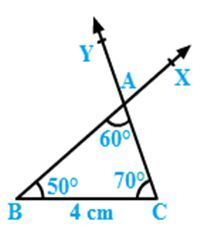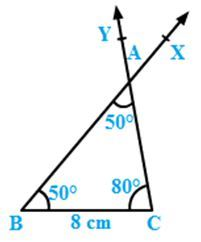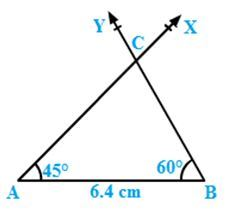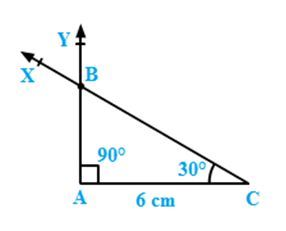×#### Thank you for registering.

One of our academic counsellors will contact you within 1 working day.

Click to Chat

1800-1023-196

+91-120-4616500

CART 0

• 0

MY CART (5)

Use Coupon: CART20 and get 20% off on all online Study Material

ITEM
DETAILS
MRP
DISCOUNT
FINAL PRICE
Total Price: Rs.

There are no items in this cart.
Continue Shopping• Complete JEE Main/Advanced Course and Test Series
• OFFERED PRICE: Rs. 15,900
• View Details

```Constructions Exercise 17.5

Question: 1

Draw a right triangle with hypotenuse of length 5 cm and one side of length 4 cm.

Solution:Steps of construction:

Draw a line segment QR = 4 cm.

Draw ∠QRX of measure 90°.

With centre Q and radius PQ = 5 cm, draw an arc of the circle to intersect ray RX at P.

Join PQ to obtain the desired triangle PQR.

PQR is the required triangle.

Question: 2

Draw a right triangle whose hypotenuse is of length 4 cm and one side is of length 2.5 cm.

Solution:Steps of construction:

Draw a line segment QR = 2.5 cm.

Draw ∠QRX of measure 90°.

With centre Q and radius PQ = 4 cm, draw an arc of the circle to intersect ray RX at P.

Join PQ to obtain the desired triangle PQR.

PQR is the required triangle.

Question: 3

Draw a right triangle having hypotenuse of length 5.4 cm, and one of the acute angles of measure 30°

Solution:Let ABC be the right triangle at A such that hypotenuse BC = 5.4 cm. Let cC = 30°.

Therefore ∠A + ∠B + ∠C = 180°∠B = 180°− 30°− 90° = 60°

Steps of construction:

Draw a line segment BC = 5.4 cm.

Draw angle CBY = 60°

Draw angle BCX of measure 30° with X on the same side of BC as Y.

Let BY and CX intersect at A.

Then ABC is the required triangle.

Question: 4

Construct a right triangle ABC in which AB = 5.8 cm, BC = 4.5 cm and ∠C = 90°.

Solution:Steps of construction:

Draw a line segment BC = 4.5 cm.

Draw ∠BCX of measure 90°..

With centre B and radius AB = 5.8 cm, draw an arc of the circle to intersect ray BX at A.

Join AB to obtain the desired triangle ABC.

ABC is the required triangle.

Question: 5

Construct a right triangle, right angled at C in which AB = 5.2 cm and BC= 4.6 cm.

Solution:Steps of construction:

Draw a line segment BC = 4.6 cm.

Draw ∠BCX of measure 90°

With centre B and radius AB = 5.2 cm, draw an arc of the circle to intersect ray CX at A.

Join AB to obtain the desired triangle ABC.

ABC is the required triangle.
```### Course Features

• 728 Video Lectures
• Revision Notes
• Previous Year Papers
• Mind Map
• Study Planner
• NCERT Solutions
• Discussion Forum
• Test paper with Video Solution